# The moons dataset and decision surface graphics in a Jupyter environment – II – contourplots

I proceed with my present article series on the “moons dataset” as an example for classification tasks in the field of “machine learning” [ML]. My objective is to gather basic knowledge on Python related tools for performing related experiments. In my last blog article

The moons dataset and decision surface graphics in an Jupyter environment – I

In the case of the “moons dataset” we can apply and train support vector machines [SVM] algorithms for solving the classification task: The trained algorithm will predict to which of the 2 clusters a new data point probably belongs. The basic task for this kind of information reduction is to find a (curved) decision surface between the data clusters in the n-dimensional representation space of the data points during the training of the algorithm.

As the moons feature space is only 2-dimensional the decision surface would be a curved line. Of course, we would like to add this line to the 2D-plot of the moons clusters shown in the last article.

The challenge of plotting data points and decision surfaces for our moon clusters

1. is sufficiently simple for a Python- and AI/ML-beginner as me,
2. is a good opportunity to learn how to work with a Jupyter notebook,
3. gives us a reason to become acquainted with some basic plotting functions of matplotlib,
4. an access to some general functions of SciKit – and some specific ones for SVM-problems.

Much to learn from one little example. Points 2 and 3 are the objectives of this article.

# Contour plots !

But what kind of plots should we be interested in? We need to separate areas of a 2-dimensional parameter space (x1,x2) for which we get different (integer) target or y-values, i.e. to distinguish between a set of distinct classes to which data points may belong – in our case either to a class “0” of the first moon like cluster and a class “1” for data points around the second cluster.

In applied mathematics there is a very similar problem: For a given function z(x1,x2) we want to visualize regions in the (x1,x2)-plane for which the z-values cover a range between 2 selected distinct z-values, so called contour areas. Such contour areas are separated by contour lines. Think of height lines in a map of a mountain region. So, there is an close relation between a contour line and a decision surface – at least in a two dimensional setup. We need contour plots!

Let us see how we start a Jupyter environment and how we produce nice 2D- and even 3D-contour-plots.

# Starting a Jupyter notebook from a virtual Python environment on our Linux machine

I discussed the setup of a virtual Python environment (“virtualenv”) already in the article Eclipse, PyDev, virtualenv and graphical output of matplotlib on KDE – I of this blog. I refer to the example and the related paths there. The “virtualenv” has a name of “ml1” and is located at “/projekte/GIT/ai/ml1”.

In the named article I had also shown how to install the Jupyter package with the help of “pip3” within this environment. You can verify the Jupyter installation by having a look into the directory “/projekte/GIT/ai/ml1/bin” – you should see some files “ipython3” and “jupyter” there. I had also prepared a directory
“/projekte/GIT/ai/ml1/mynotebooks”
to save some experimental notebooks there.

How do we start a Jupyter notebook? This is simple – we just use a
terminal window and enter:

```myself@mytux:/projekte/GIT/ai/ml1> source bin/activate
(ml1) myself@mytux:/projekte/GIT/ai/ml1> jupyter notebook
[I 16:16:29.040 NotebookApp] Serving notebooks from local directory: /projekte/GIT/ai/ml1
[I 16:16:29.040 NotebookApp] The Jupyter Notebook is running at:
[I 16:16:29.040 NotebookApp] http://localhost:8888/?token=942e6f5e75b0d014659aea047b1811d1992ca77e4d8cc714
[I 16:16:29.040 NotebookApp] Use Control-C to stop this server and shut down all kernels (twice to skip confirmation).
[C 16:16:29.054 NotebookApp]

To access the notebook, open this file in a browser:
file:///run/user/1004/jupyter/nbserver-19809-open.html
Or copy and paste one of these URLs:
http://localhost:8888/?token=942e6f5e75b0d014659aea047b1811d1992ca77e4d8cc714
```

We see that a local http-server is started and that a http-request is issued. In the background on my KDE desktop a new tag in my standard browser “Firefox” is opened for this request: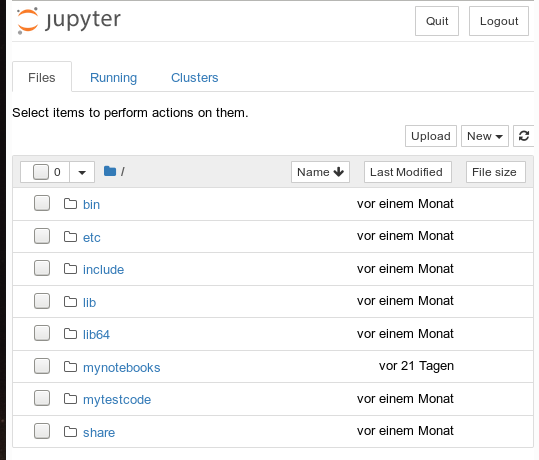Note that a standard port 8888 is used; this port should not be used by other services on your machine.

On the displayed web page we can move to the “mynotebooks” directory. We open a new notebook there by clicking on the “New”-button on the right side of the browser window:

We choose Python3 as the relevant interpreter and get a new browser window: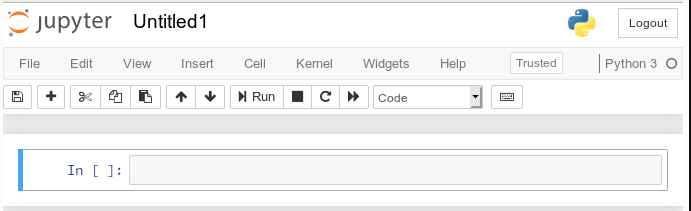We give the notebook a title by clicking on “File >> Save as …” before start using the provided input “cell” for coding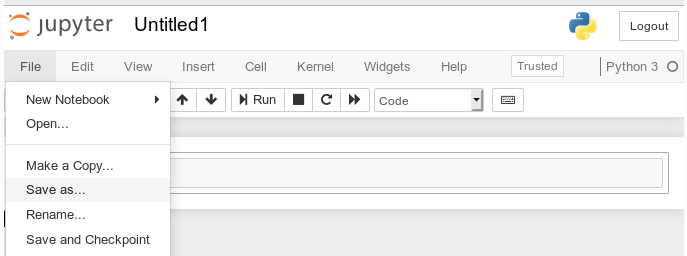I name it “moons1” in the next input form and check afterward in a terminal that the file “/projekte/GIT/ai/ml1mynotebooks/moons1.ipynb” really has been created; you see this also in the address bar of the browser – see below.

# Lets do some plotting within a notebook

Most of the icons regarding the notebook screen are self explanatory. The interesting and pretty nice thing about a Jupyter notebook is that the multiple lines of Python code can be filled into cells. All lines can be executed in a row by first choosing a cell via clicking on a it and then clicking on the “Run” button.

As a first exercise I want to do some plotting with “matplotlib” (which I also installed together with the numpy package in a previous article). We start by importing the required modules: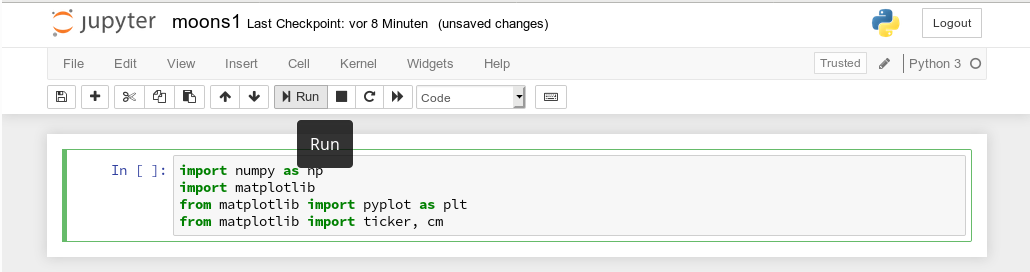A new cell for input opens automatically (it is clever to separate cells for imports and for real code). Let us produce a most simple plot there: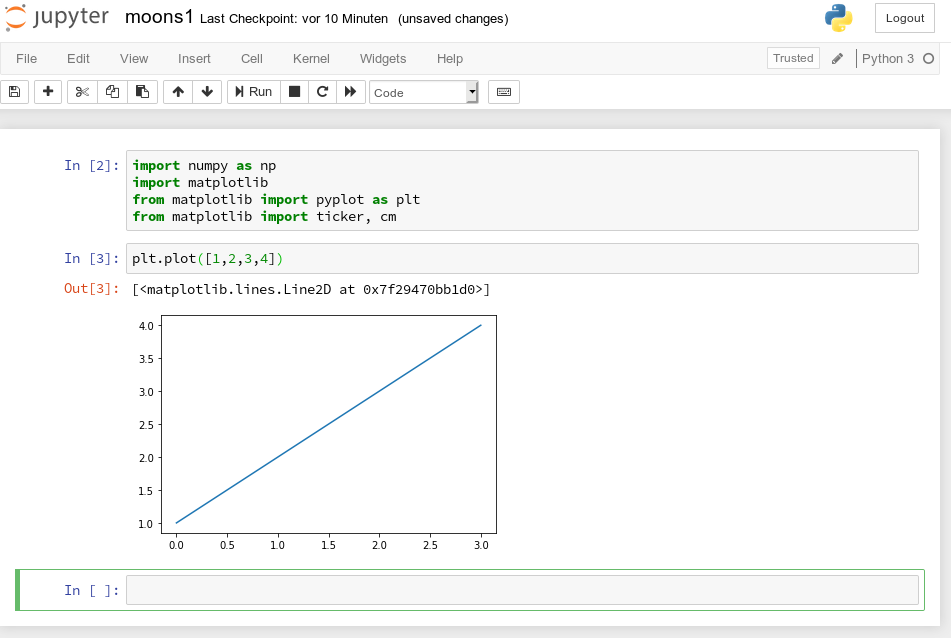No effort in comparison to what we had to do to prepare an Eclipse environment for plotting (see Eclipse, PyDev, virtualenv and graphical output of matplotlib on KDE – II). Calling plot routines simply works – no special configuration is required. Jupyter and the browser do all the work for us. We save our present 2 cells by clicking on the “Save“-icon.

# How do we plot contour lines or contour areas?

Later on we need to plot a separation line in a 2-dimensional parameter space between 2 clustered sets of data. This task is very similar to plotting a contour line. As this is a common task in math we expect matplotlib to provide some functionality for us. Our ultimate goal is to wrap this plotting functionality into a function or class which also accepts an SVM based ML-method of SciKit to prepare and evaluate the basic data first. But let us proceed step by step.

Some research on the Internet shows: The keys to contour plotting with matplotlib are the functions “contour()” and “contourf()” (matplotlib.pyplot.contourf):

contour(f)([X, Y,] Z, [levels], **kwargs)

“contour()” plots lines, only, whilst “contourf()” fills the area between the lines with some color.

Both functions accept data sets in the form of X,Y-coordinates and Z-values (e.g. defined by some function Z=f(X,Y)) at the respective points.

X and Y can be provided as 1-dim arrays; Z-values, however, must be given by a 2-dim array, such that len(X) == M is the number of columns in Z and len(Y) == N is the number of rows in Z. We cover the X,Y-plane with Z-values from bottom to top (Y, lines) and from the left to the right (X, columns).

Somewhat counter-intuitively, X and Y can also be provided as 2-dim arrays – with the same dimensionality as Z.
There is a nice function “meshgrid” (of packet numpy) which allows for the creation of e.g. a mesh of two 2-dimensional X- and separately Y-matrices. See for further information (numpy.meshgrid). Both arrays then have a (N,M)-layout (shape); as the degree of information of one coordinate is basically 1-dimensional, we do expect repeated values of either coordinate in the X-/Y-meshgrid-matrices.

The function “shape” gives us an output in the form of (N lines, M columns) for a 2-dim array. Lets apply all this and create a rectangle shaped (X,Y)-plane: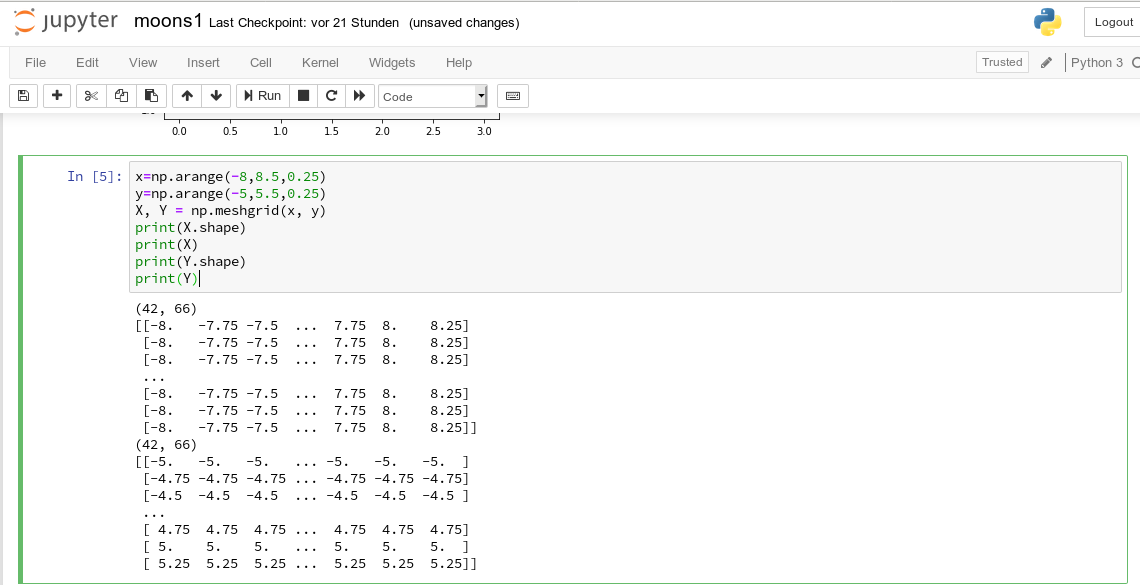The basic numpy-function “arange()” turns a range between two limiting values into an array of equally spaced values. We see that meshgrid() actually produces two 2-dim arrays of the same “shape”.

For test purposes let us use a function

Z1=-0.5* (X)**2 + 4*(Y)**2.

For this function we expect elliptical contours with the longer axis in X-direction. The “contourf()”-documentation shows that we can use the parameters “levels“, “cmap” and “alpha” to set the number of contour levels (= number of contour lines -1), a so called colormap, and the opacity of the area
coloring, respectively.

You find predefined colormaps and their names at this address: matplotlib colormaps. If you add an “_r” to the colormap-name you just reverse the color sequence.

We combine all ingredients now to create a 2D-plot (with the “plasma” colormap):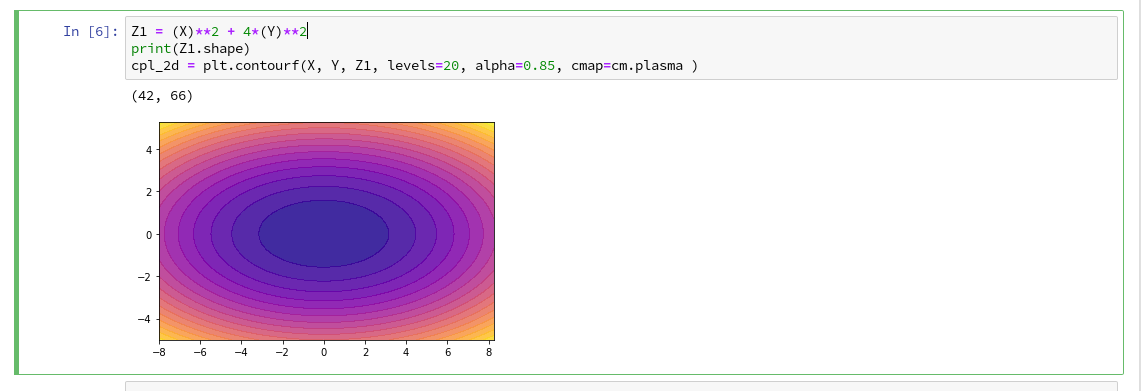Our first reasonable contour-plot within a Jupyter notebook! We got the expected elliptic curves! Time for a coffee ….

# Changing the plot size

A question that may come to your mind at this stage is: How can we change the size of the plot?

Well, this can be achieved by defining some basic parameters for plotting. You need to do this in advance of any of your specific plots. One also wants to add some labels for all axis. We, therefore, extend the code in our cell a bit by the following statements and click again on “Run”:You see that “fig_size = plt.rcParams[“figure.figsize”]” provides you with some kind of array- or object like information on the size of plots. You can change this by assigning new values to this object. “figure” is an instance of a container class for all plot elements. “plt.xlabel” and “plt.ylabel” offer a simple option to add some text to an axis of the plot.

# What about a 3D-representation …

As we are here – isn’t our function for Z1 not a good example to get a 3D-representation of our data? As 3D-plots are helpful in other contexts of ML, lets have a quick side look at this. You find some useful information at the following addresses:
PythonDataScienceHandbook and mplot3d-tutorial

I used the given information in form of the following code: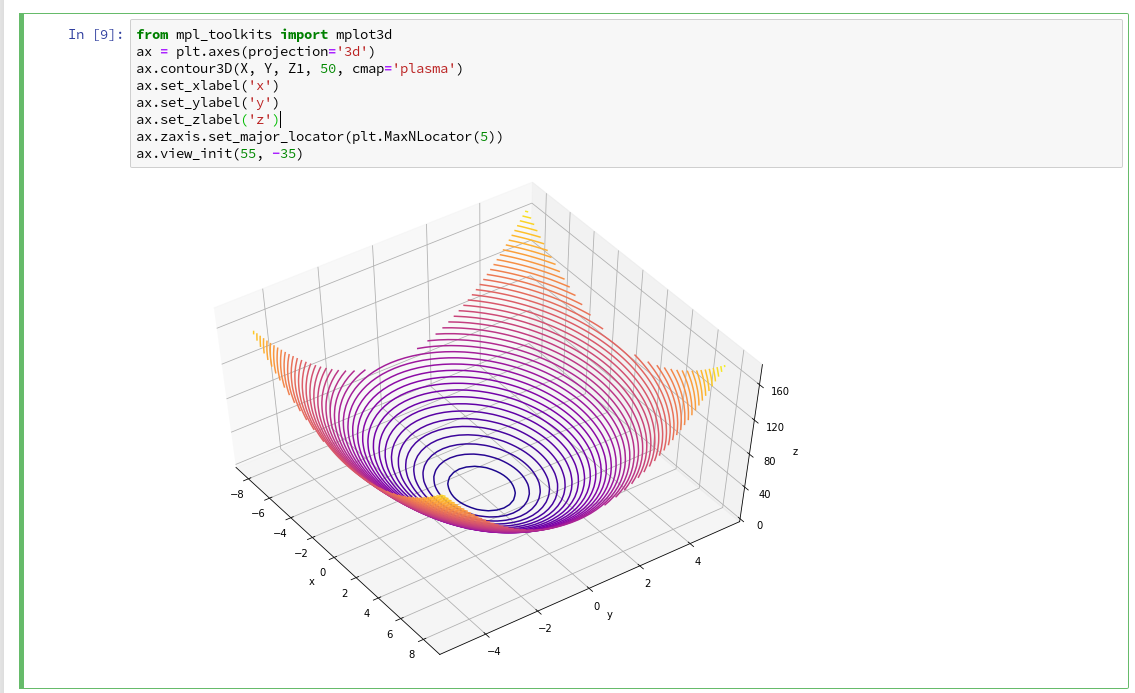You see that we can refer to a special 3D-plot-object as the output of plt.axes(projection=’3d’). The properties of such an object can be manipulated by a variety of methods. You also see that I manipulated the number of ticks on the z-axis to 5 by using a function “set_major_locator(plt.MaxNLocator(5)“. I leave it to the reader to dive deeper into manipulation options for a plot axis.

A reader asked me to show how one can set ticks and add a color-bar to the plots. I give an example code below:The result is: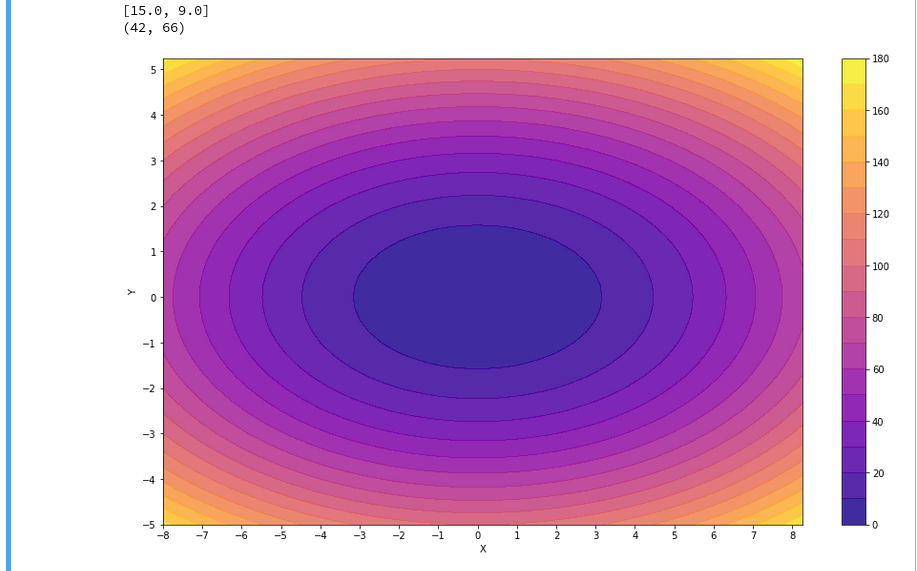For the 3D-plots we get:# Conclusion

Enough for today. We have seen that it is relatively simple to create nice contour and even 3D-plots in a Jupyter notebook environment. This new knowledge provides us with a good basis for a further approach to our objective of plotting a decision surface for the moons dataset. In the next article

The moons dataset and decision surface graphics in a Jupyter environment – III – scatter-plots and LinearSVC

we first import the moons data set into our Jupyter notebook. Then we shall create a so called “scatter plot” for all data points. Furthermore we shall train a specific SVM algorithm (LinearSVC) on the dataset.

|

# The moons dataset and decision surface graphics in a Jupyter environment – I

For me as a beginner in Machine Learning [ML] and Python the first interesting lesson was that artificial neural networks are NOT needed to solve various types of problems. A second lesson was that I needed a lightweight tool for quick experiments in addition to Eclipse PyDev – which lead me to Jupyter notebooks. A third lessons was that some fundamental knowledge about plotting functionalities provided by Sklearn is mandatory – especially in the field of classification tasks, where you need to visualize decision or separation surfaces. For a newbie both in ML, Python and SciKit/Sklearn the initial hurdles are many and time consuming. Not all text books were really helpful – as the authors often avoid a discussion of basic code elements on a beginner level.

Playing around with a special test and training example for beginners – namely the moons data set – helped me to overcome initial obstacles and to close some knowledge gaps. With this article series I want to share some of my insights with other people starting to dive into the field of ML. ML-professionals will not learn anything new.

# Classification, SVM and the moons dataset

An interesting class of ML-problems is the reduction of information in the following sense:

A set of data vectors describes data points in a n-dimensional representation space. Each individual data point in the set belongs to a specific cluster of multiple existing disjunctive clusters of data points in the representation space. After a training an algorithm shall predict for any new given data point vector to which cluster the data point belongs.

This poses a typical classification task in ML. I talk of a representation space as it represents the “n” different features of our data points. The representation space is sometimes also called “feature space”.

“Disjunctive” is to be understood in a geometrical sense: The regions the clusters occupy in the representation space should be clearly separable. A “separation surface” – mathematically a hyperplane – should exist which separates two clusters from each other.

Each cluster may exhibit special properties of its members. Think e.g. of diseases; a certain combination of measured parameter or “feature” values describing the status of a patient’s body (temperature, blood properties, head ache, reduced lung capacity, ..) may in a complicated way decide between different kinds of virus infections.

In such a case an ML-algorithm – after a training – has to separate the multidimensional representation space into disjunctive regions and afterward determine (by some exact criteria) into which region the “feature” values in some given new data vector place the related data point. To achieve this kind of prediction capability may in real world problems be quite challenging:

The data points of each known cluster may occupy a region with a complex curved or even wiggled surface in the representation space. Therefore, most often the separation surface of data point clusters can NOT be described in a simple linear way; the separation hyperplane between clusters in a multidimensional representation space is typically given by a non-linear function; it describes a complex surface with a dimensionality of n-1 inside the original n-dim vector space for data representation.

One approach to find such hyperplanes was/is e.g. the use of so called “Support Vector Machines” (as described e.g. in chapter 5 of the brilliant book of “Machine Learning with SciKit-Learn & TensorFlow” by A. Geron; published by O’Reilly, 2018).

An interesting simple example for using the SVM approach is the “moon” dataset. It displays 2 disjunctive clusters of data in a 2-dimensional
representation space ( with coordinates x1 and x2 for two features). The areas are formed like 2 moon crescents.

The 2-dim vectors describing the data points are associated with target values of “0” and “1” – with “0” describing the membership in the upper cluster and “1” the membership in the lower cluster of points. We see at once that any hyperplane – in this case a one dimensional, but curved line – separating the data clusters requires some non-linear description with respect to x1 and x2.

We also understand that we need some special plotting technique to visualize the separation. Within the named book of Geron we do not find a recipe for such a plot; however you do find example programs on the author’s website for the exercises in the book. Actually, I found some additional hints in a different book “Python Machine Learning” of Sebastian Raschka, pubished by PACKT Publishing, 2016. When I came to Geron’s discussion of the moon dataset I tried to combine it with the plotting approach of Raschka. For me – as a beginner – the whole thing was a very welcome opportunity to do some exercises with a Jupyter notebook and write some small functions for plotting decision regions.

I describe my way of dealing with the moon dataset below – and hope it may help others. However, before we move to Jupyter based experiments I want to give some hints on the SVM approach and Python libraries required.

# SVM, “Polynomial Features” and the SVC Kernel

The algorithm behind a SVM performs an analysis of data point vectors in a multidimensional vector space and the distances of the points of different clusters. The strategy to find a separating hyperplane between data point clusters should follow 3 principles:

• We want to guarantee a large separation in terms of metrical distances of outer cluster points to the separation plane.
• We want to minimize the number of of points violating the separation.
• The number of mathematical operations shall be reduced.

If we had some vector analysis a part of our education we may be able to figure out how to determine a hyperplane in cases where a linear separation is sufficient. We adjust the parameters of the linear hyperplane such that for some defined average the distance of carefully chosen cluster points to the plane is maximized. We could also say that we try to “maximize” the margins of the data clusters to the hyperplane (see the book of Raschka, cahpter 3, around page 70). We can moderate the “maximize” strategy by some parameters allowing for a narrower margin not to get too many wrongly placed data points. Thus we would avoid overfitting. But what to do about a non-linear separation surface?

Non-linearity can be included by a trick: Polynomial variations of the data variables – i.e. powers of the data variables – are added as new so called slack variables to the original data sets – thereby extending the dimensionality of the sets. In the extended new vector space data which previously were not separable can appear in clearly separated regions.

A SciKit-Learn Python class “PolynomialFeatures” supports variations in the combination of powers of the original data up to a limiting total power (e.g. 3). If the 2 dim data variables are x1 and x2, we may e.g. include x1**3, x2**3, x2*x1**2, x1*x2**2 and x1*x2 as new variables. On the extended vector space we could then apply a conventional linear analysis again.

Such a linear analysis is provided by a Python module called LinearSVC which is part of “sklearn.svm”, which provides SVM
related classes and methods. PolynomialFeatures is a class and part of “sklearn.preprocessing”.

Note that higher polynomials obviously will lead to a steep rise in the number of data operations as the dimensionality of the data sets grows significantly due to combinatorics. For many cases it can, however, be shown that it is sufficient to operate with the original data points in the lower dimensional representation space in a special way to cover polynomial extensions or distance calculations for the hyperplane determination in the higher dimensional and extended representation space. A so called “Polynomial Kernel” function takes care of this without really extending the dimensionality of the original datasets.

Note that there are other kernel functions for other types of calculation approaches to determine the separation surface: One approach uses an exponentially weighted distance (Gaussian kernel or RBF-kernel) between points in the sense of a similarity function to add new features (= dimensions) to the representation space. In this case we have to perform mathematical operations on pairs of data points in the original space with lower dimensionality, only.

Thus, different kinds of kernel functions are included in a SciKit-Learn class named “SVC“. All methods work sufficiently well at least for small and medium data sets. For large datasets the polynomial Kernel may scale badly regarding CPU time.

There is another kind of scaling – namely of the data to reasonable value regions. It is well known that data scaling is important when using SVM for the determination of decision surfaces. So, we need another Python class to scale our “moon” data reasonably – “StandardScaler” which is part of sklearn.preprocessing.

# Required Python libraries

We assume that we have installed the Python packets sklearn, mathlotlib and numpy in the virtual Python environment for our ML experiments, already. (See previous articles in this blog for the establishment of such an environment on a Linux machine.)

We shall use the option of SciKit-Learn to build pipelines of operations on datasets. So, we need to import the class “Pipeline” from sklearn.pipeline. The “moons dataset” itself is part of the datasets library of “sklearn”.

All in all we will use the following libraries in the forthcoming experiments:

```import numpy as np
import matplotlib.pyplot as plt
from matplotlib.colors import ListedColormap

from sklearn.datasets import make_moons

from sklearn.pipeline import Pipeline
from sklearn.preprocessing import StandardScaler
from sklearn.preprocessing import PolynomialFeatures

from sklearn.svm import LinearSVC
from sklearn.svm import SVC
```

Enough for today.In the next article

The moons dataset and decision surface graphics in a Jupyter environment – II – contourplots

we will acquire some basic tool skills in ML: I shall show how to start a Jupyter notebook and how to create contour-plots. We will need this knowledge later on for doing experiments with our moons data set and to visualize the results of SVM algorithms for classification.

|